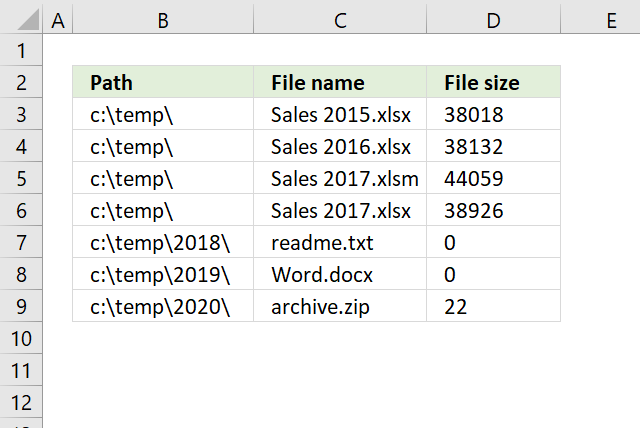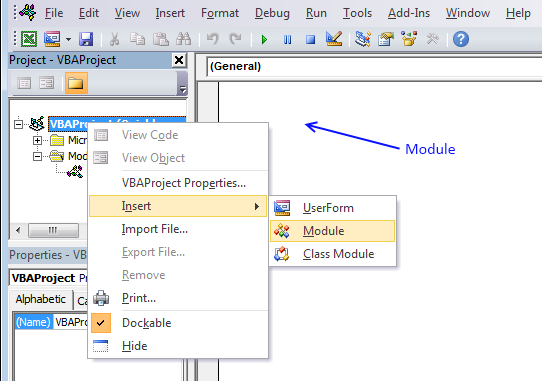Author: Oscar Cronquist Article last updated on January 24, 2019This article demonstrates a user defined function that lists files in a ggiven folder and subfolders. A user defined function is a custom function in Excel that anyone can build, simply copy the VBA code and paste to your workbook's code module and you are good to go.

Array formula in cell range B3:D9:

=ListFiles("c:\temp")

To enter an array formula, select the cell range, type the formula, then press and hold CTRL + SHIFT simultaneously, now press Enter once. Release all keys.

The formula bar now shows the formula with a beginning and ending curly bracket telling you that you entered the formula successfully. Don't enter the curly brackets yourself.

### User defined Function Syntax

ListFiles(FolderPath)

### Arguments

 Parameter Text FolderPath Required. The path to the folder you want to use.

### Where to copy vba code?1. Press Alt-F11 to open visual basic editor
2. Click Module on the Insert menu
3. Copy and paste vba code, both functions below.
4. Exit visual basic editor
```'Declare a public variable meaning it will exist for both functions below.
Public temp() As String

'Name function and declare parameters
Function ListFiles(FolderPath As String)
'Declare arguments and data types
Dim k As Long, i As Long
'Redimension array variable temp so it can contain more values if needed.
ReDim temp(2, 0)
'Add and ending backslash to pathe if missing
If Right(FolderPath, 1) <> "\" Then
FolderPath = FolderPath & "\"
End If
'Start second function named Recursive with folder path
Recursive FolderPath
'Count cells the UDF is using
k = Range(Application.Caller.Address).Rows.Count
'Return a message if cell range needs to be larger in order to show all values
'or fill array values with blanks to avoid error values when no more values is to be shown
If k < UBound(temp, 2) Then
MsgBox "There are more rows, extend user defined function"
Else
For i = UBound(temp, 2) To k
ReDim Preserve temp(UBound(temp, 1), i)
temp(0, i) = ""
temp(1, i) = ""
temp(2, i) = ""
Next i
End If
'Return values
ListFiles = Application.Transpose(temp)
ReDim temp(0)
End Function
```
```'Name function and declare parameter
Function Recursive(FolderPath As String)
'Declare variables and data types
Dim Value As String, Folders() As String
Dim Folder As Variant, a As Long
'Redimension array variable Folders so it can contain more folder paths if needed.
ReDim Folders(0)
'If folder path ends with \\ then end function
If Right(FolderPath, 2) = "\\" Then Exit Function
'Open folder path and populate Value with file names/folders
Value = Dir(FolderPath, &H1F)
'Repeat code until all file names have been retrieved
Do Until Value = ""
'Ignore file name . and ..
If Value = "." Or Value = ".." Then
Else
'If value is a folder then save value to variable Folders
If GetAttr(FolderPath & Value) = 16 Then
Folders(UBound(Folders)) = Value
ReDim Preserve Folders(UBound(Folders) + 1)
Else
'If value is a file name then save path, name and size to variable temp
temp(0, UBound(temp, 2)) = FolderPath
temp(1, UBound(temp, 2)) = Value
temp(2, UBound(temp, 2)) = FileLen(FolderPath & Value)
ReDim Preserve temp(UBound(temp, 1), UBound(temp, 2) + 1)
End If
End If
'Continue with next value in folder
Value = Dir
Loop
'Start a new instance of function Recursive for each new folder
For Each Folder In Folders
Recursive FolderPath & Folder & "\"
Next Folder
End Function
```

### Download Excel file

* You will also get a weekly newsletter, unsubscribe anytime!